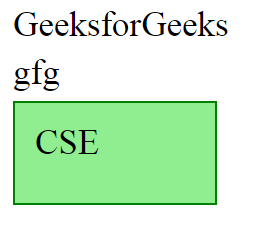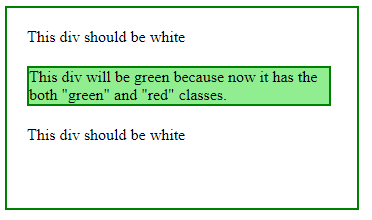Open In App
Related Articles

The addClass() method is an inbuilt method in jQuery that is used to add more properties to each selected element. It can also be used to change the property of the selected element.

This method can be used in two different ways:
1) By adding a Class name directly: Here, the Class name can be used directly with the element which is going to be selected.

Syntax:

`\$(selector).addClass(className);`

Parameters: It accepts the parameter “className” which is the name of the class that is going to be added.

Return Value: It returns the selected elements with the added new class.

Example 1: In this example, we are using the above-explained method.

## HTML

 ```<``html``>` `<``head``>``    ``<``meta` `charset``=``"utf-8"``>``    ``<``script` `src``=``"https://code.jquery.com/jquery-1.10.2.js"``>``    ````    ``<``title``>addClass demo``    ``<``style``>``        ``p {``            ``margin: 8px;``            ``font-size: 35px;``        ``}` `        ``.selected {``            ``display: block;``            ``border: 2px solid green;``            ``width: 160px;``            ``height: 60px;``            ``background-color: lightgreen;``            ``padding: 20px;``        ``}` `        ``.highlight {``            ``background: yellow;``        ``}``    ````` `<``body``>``    ``<``p``>GeeksforGeeks``    ``<``p``>gfg``    ``<``p``>CSE``    ``<``script``>``        ``\$("p").last().addClass("selected");``    ````` ``

In the above code, “p” element is selected, and the “selected” class is applied only to the last “p” element with the help of .last() method and .addClass() method of jQuery.

Output:2) By passing a function to add a new class: Here, A function can be passed to the .addClass() for the selected element.

Syntax:

`\$(selector).addClass(function);`

Parameters: It accepts a parameter “function”.

Return Value: It returns the selected element with added function.

Example 2:  Here is the example of the above-explained method.

## HTML

 ```<``html``>` `<``head``>``    ``<``meta` `charset``=``"utf-8"``>``    ``<``script` `src``=``"https://code.jquery.com/jquery-1.10.2.js"``>``    ````    ``<``style``>``        ``div {``            ``background: white;``            ``margin: 20px;``        ``}` `        ``.red {``            ``background: red;``            ``width: 300px;``            ``margin: 20px;``        ``}` `        ``.red.green {``            ``background: lightgreen;``            ``margin: 20px;``            ``border: 2px solid green;``        ``}` `        ``body {``            ``border: 2px solid green;``            ``width: 350px;``            ``height: 200px;``        ``}``    ````` `<``body``>``    ``<``div``>This div should be white``    ``<``div` `class``=``"red"``>``        ``This div will be green because now it``        ``has the both "green" and "red" classes.``    ````    ``<``div``>This div should be white` `    ``<``script``>``        ``\$("div").addClass(function (index, currentClass) {``            ``let addedClass;``            ``if (currentClass === "red") {``                ``addedClass = "green";``                ``\$("p").text("There is one green div");``            ``}``            ``return addedClass;``        ``});``    ````` ``

In the above code, the “div” element is selected and with the help of a function, a new class is added to the selected div element.

Output: